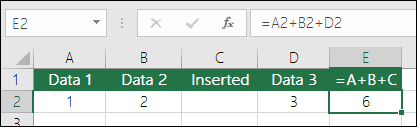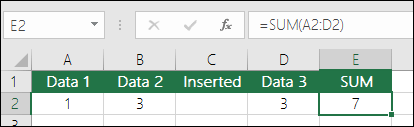# SUM Function – Overview

This function simply adds values; whether single, cell references/ranges or a combination of all three.

For example:

• =SUM(B2+B3+B4+B5) – Adds each individual cell from B2 to B5.
• =SUM(B2:B5) – Adds the values in the cell range of B2:B5.

## Syntax:

SUM(number1,[number2],…)

## Best Practices with SUM

This section will discuss some best practices for working with the SUM function. Much of this can be applied to working with other functions as well.

The =1+2 or =A+B Method – While you can enter =1+2+3 or =A1+B1+C2 and get fully accurate results, these methods are error prone for several reasons:

## Typos

1. Typos – Imagine trying to enter more and/or much larger values like this:

• =14598.93+65437.90+78496.23

Then proceed to validate that your contents are correct. It’s considerably simpler to place these values in separate cells and use a SUM formula. Also, you can format the values once they’re among cells, increasing their legibility as soon as they’re in a formula.

## #VALUE! errors from referencing text instead of numbers

1. #VALUE! errors from referencing text instead of numbers

If you apply a formula like:

=A1+B1+C1 or =A1+A2+A3

Your formula can malfunction if there are any non-numeric (text) values in the referenced cells, which generates a #VALUE! error. SUM skips text values and provides you with the sum of only the numeric values.

### #REF! error from deleting rows or columns

1. #REF! error from deleting rows or columns

If you delete a row or column, the formula will not update to exclude the deleted row and it will return a #REF! error, where a SUM function will automatically update.### Formulas won’t update references when inserting rows or columns

1. Formulas won’t update references when inserting rows or columnsIf you add a row or column, the formula will remain static after rows or columns are added, where a SUM function will instantly update (as long as you’re not outside of the range referenced in the formula). This is particularly vital if you anticipate your formula to become revised and it doesn’t, since it will leave you with partial results that you might overlook.### SUM with individual Cell References vs. Ranges

1. SUM with individual Cell References vs. Ranges

Using a formula like:

• =SUM(A1,A2,A3,B1,B2,B3)

Is similarly error prone during adding or erasing rows within the referenced range for the same rationales. It’s more effective to apply individual ranges, like:

• =SUM(A1:A3,B1:B3)

Which will update when adding or deleting rows.

## Question 1

1. I just want to Add/Subtract/Multiply/Divide numbers – See this video series on Basic Maths in Excel, or Use Excel as your calculator.

## Question 2

1. How do I show more/less decimal places? You can edit your number format. Choose the cell or range in question and use Ctrl+1 to launch the Format Cells Dialogue, then pick the Number tab and decide your preferred format, ensuring to reveal your desired number of decimal places.

## Question 3

1. How do I add or subtract Times? You can add and subtract times in a few different ways. For example, to find the difference between 8:00 AM – 12:00 PM for payroll purposes you would use: =(“12:00 PM”-“8:00 AM”)*24, taking the end time minus the start time. Note that Excel calculates times as a fraction of a day, so you must multiply by 24 to obtain the total hours. In the first example, we’re using =((B2-A2)+(D2-C2))*24 to attain the sum of hours from start to finish, less a lunch break (8.50 hours total).
1. If you’re merely adding hours and minutes and want to display that way, then you can sum without having to multiply by 24, so in the second example, we’re using =SUM(A6:C6) as we only require the total number of hours and minutes for assigned tasks (5:36, or 5 hours, 36 minutes).

## Question 4

1. How do I get the difference between dates? As with times, you can add and subtract dates. Here’s a very frequent example of counting the number of days between two dates. It’s as simple as =B2-A2. The key to working with both Dates and Times is that you start with the End Date/Time and subtract the Start Date/Time.

For more ways to work with dates see: Calculate the difference between two dates.

## Question 5

1. How do I sum just visible cells? Sometimes, when you physically hide rows or use AutoFilter to present merely specific data you also just want to sum the visible cells. You can use the SUBTOTAL function. If you’re using a total row in an Excel table, any function you pick from the Total drop-down will promptly be added as a subtotal. See more about how to Total the data in an Excel table.

## Need more help?

You can always ask an expert in the Excel Tech Community, get support in the Answers community, or suggest a new feature or improvement on Excel User Voice.

The SUMIF function adds only the values that meet a single criteria

The SUMIFS function adds only the values that meet multiple criteria

The COUNTIF function counts only the values that meet a single criteria

The COUNTIFS function counts only the values that meet multiple criteria

Overview of formulas in Excel

How to avoid broken formulas

Find and correct errors in formulas

Common formula problems and how to fix them

Maths & Trig functions

Excel functions (alphabetical)

Excel functions (by Category)